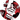# How to calculate the number of days between 2 dates in Python?

February 05, 2021

In this article, you will learn how to calculate the number of days between 2 dates in Python.

At the beginning, import `date` from `datetime`..

``from datetime import date``

Then, define the first and second date

``````first_date = date(2021, 1, 31)
second_date = date(2021, 2, 28)``````

Then, calculate `second_date` - `first_date`

``difference = second_date - first_date``

At the end, print `difference.days`

``print(difference.days) # 28``Written by Murtaja Ziad, a full-stack web developer.
My website - Follow me on Twitter